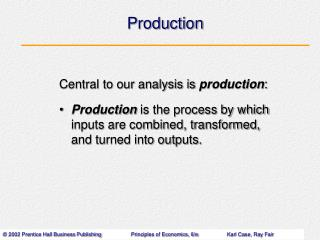DownloadDownload PresentationProduction

# Production

Download Presentation## Production

- - - - - - - - - - - - - - - - - - - - - - - - - - - E N D - - - - - - - - - - - - - - - - - - - - - - - - - - -
##### Presentation Transcript

1. Production Central to our analysis is production: • Production is the process by which inputs are combined, transformed, and turned into outputs.

2. What Is A Firm? • A firm is an organization that comes into being when a person or a group of people decides to produce a good or service to meet a perceived demand. Most firms exist to make a profit. • Production is not limited to firms.

3. Perfect Competition • many firms, each small relative to the industry, • producing virtually identical products and • in which no firm is large enough to have any control over prices. • In perfectly competitive industries, new competitors can freely enter and exit the market. Perfect competition is an industry structure in which there are:

4. Homogeneous Products • Homogeneous products are undifferentiated products; products that are identical to, or indistinguishable from, one another.

5. Competitive Firms are Price Takers • In a perfectly competitive market, individual firms are price-takers. This means that firms have no control over price. Price is determined by the interaction of market supply and demand.

6. Demand Facing a Single Firm in a Perfectly Competitive Market • If a representative firm in a perfectly competitive market rises the price of its output above \$2.45, the quantity demanded of that firm’s output will drop to zero. Each firm faces a perfectly elastic demand curve, d.

7. 1. 2. 3. How much output to supply Which production technology to use How much of each input to demand The Behavior ofProfit-Maximizing Firms • The three decisions that all firms must make include:

8. Profits and Economic Costs • Profit (economic profit) is the difference between total revenue and total cost. • Total revenue is the amount received from the sale of the product: (q X P) • Total cost (total economic cost) is the total of • Out of pocket costs, • Normal rate of return on capital, and • Opportunity cost of each factor of production.

9. Normal Rate of Return • The normal rate of return is a rate of return on capital that is just sufficient to keep owners and investors satisfied. • For relatively risk-free firms, it should be nearly the same as the interest rate on risk-free government bonds.

10. Calculating Total Revenue, Total Cost, and Profit

11. Short-Run Versus Long-Run Decisions • The short run is a period of time for which two conditions hold: • The firm is operating under a fixed scale (fixed factor) of production, and • Firms can neither enter nor exit an industry.

12. Short-Run Versus Long-Run Decisions • The long run is a period of time for which there are no fixed factors of production. Firms can increase or decrease scale of operation, and new firms can enter and existing firms can exit the industry.

13. Determining the Optimal Methodof Production • The optimal method of production is the method that minimizes cost.

14. The Production Process • Production technology refers to the quantitative relationship between inputs and outputs. • A labor-intensive technology relies heavily on human labor instead of capital. • A capital-intensive technology relies heavily on capital instead of human labor.

15. The Production Function • The production function or total product function is a numerical or mathematical expression of a relationship between inputs and outputs. It shows units of total product as a function of units of inputs.

16. Marginal Product and Average Product • Marginal product is the additional output that can be produced by adding one more unit of a specific input, ceteris paribus. • Average product is the average amount produced by each unit of a variable factor of production.

17. The Law of DiminishingMarginal Returns • The law of diminishing marginal returns states that: When additional units of a variable input are added to fixed inputs, the marginal product of the variable input declines.

18. Production Function for Sandwiches

19. Total, Average, and Marginal Product • Marginal product is the slope of the total product function. • At point A, the slope of the total product function is highest; thus, marginal product is highest. • At point C, total product is maximum, the slope of the total product function is zero, and marginal product intersects the horizontal axis.

20. Total, Average, and Marginal Product • When a ray drawn from the origin falls tangent to the total product function, average product is maximum and equal to marginal product. • Then, average product falls to the left and right of point B.

21. Total, Average, and Marginal Product • As long as marginal product rises, average product rises. • When average product is maximum, marginal product equals average product. • When average product falls, marginal product is less than average product.

22. Production Functions with Two Variable Factors of Production • In many production processes, inputs work together and are viewed as complementary. • For example, increases in capital usage lead to increases in the productivity of labor. • Given the technologies available, the cost-minimizing choice depends on input prices.

23. Production Functions with Two Variable Factors of Production